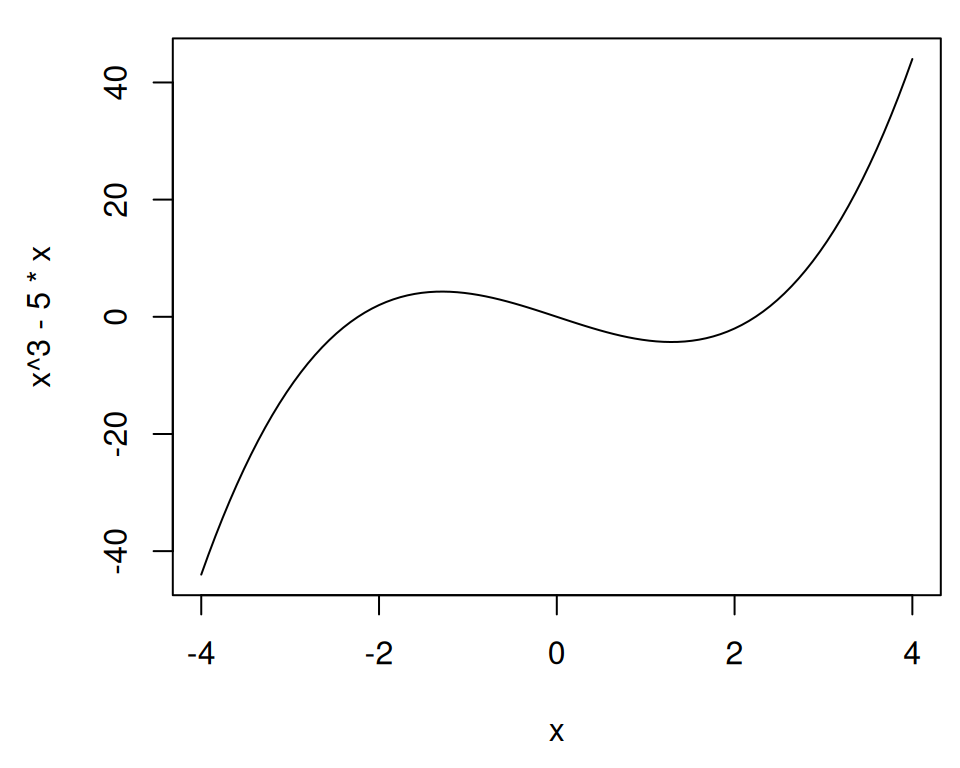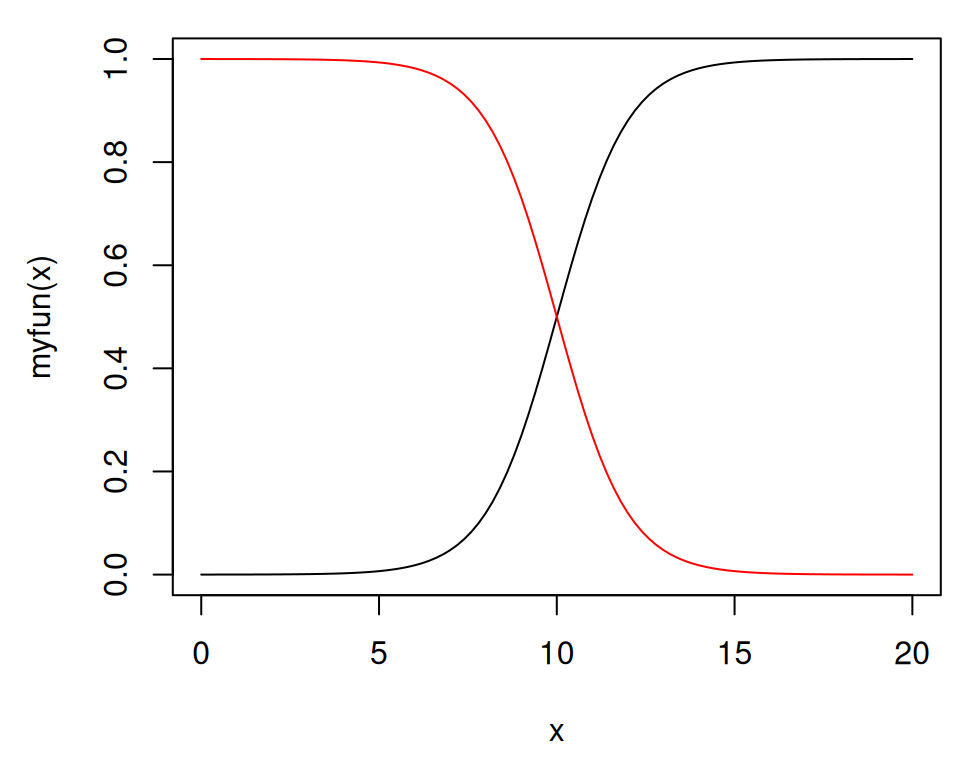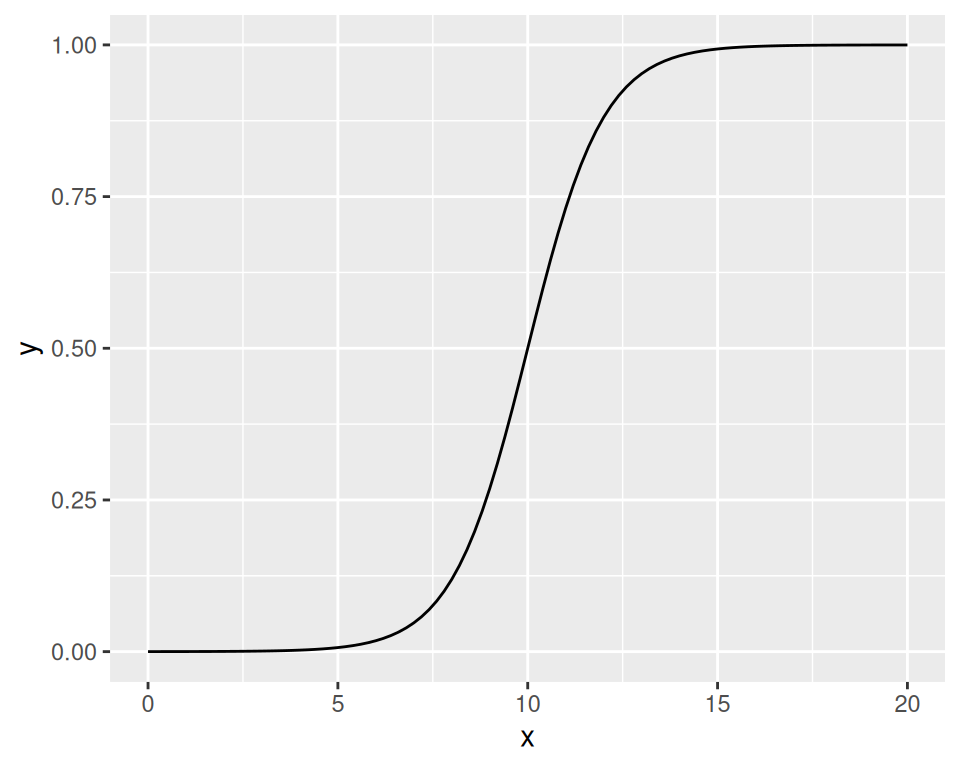## 2.6 Plotting a Function Curve

### 2.6.1 Problem

You want to plot a function curve.

### 2.6.2 Solution

To plot a function curve, as in Figure 2.12, use `curve()` and pass it an expression with the variable x:

``curve(x^3 - 5*x, from = -4, to = 4)``Figure 2.12: Function curve with base graphics (left); With user-defined function (right)

You can plot any function that takes a numeric vector as input and returns a numeric vector, including functions that you define yourself. Using `add = TRUE` will add a curve to the previously created plot:

``````# Plot a user-defined function
myfun <- function(xvar) {
1 / (1 + exp(-xvar + 10))
}
curve(myfun(x), from = 0, to = 20)
curve(1 - myfun(x), add = TRUE, col = "red")``````

With ggplot2, you can get a similar result (Figure 2.13), by using `stat_function(geom = "line")` and passing it a function that takes a numeric vector as input and returns a numeric vector:

``````library(ggplot2)
# This sets the x range from 0 to 20
ggplot(data.frame(x = c(0, 20)), aes(x = x)) +
stat_function(fun = myfun, geom = "line")``````Figure 2.13: A function curve with ggplot2